## Homework ONE

Problems 1,2 and 3 are taken from the First Edition.
1. For an infinitesimal volume, informally show that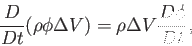where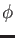is some differentiable propery of fluid. Hence informally deduce that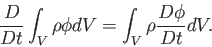2. Show that the derivative of an integral is given by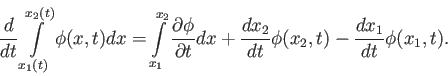By generalizing to three dimensions show that the material derivative of an integral of fluid property is given by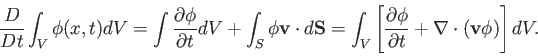where the surface integral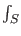is over the surface bounding the volume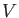. Hence show thatHint You may find it useful to Google Leibnitz's rule and Reynolds transport theorem.
3. Why is there no diffusion term in the mass continuity equation? Suppose that a fluid contains a binary mixture of dry air and water vapour. Show that the change in mass of a parcel of air due to the diffusion of water vapour is exactly balanced by the diffusion of dry air in the opposite direction.
4. Estimate the Reynolds number of flow generated by your car when you go on the North way according to the speed limit. Assume that the size of vortices generated by your car are of the order of the car cross section, nad velocity of vortices are roughly equal to the velocity of your car. The kinematic viscosity of air at 15 Centigrade is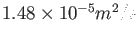. Estimate the Reynolds numbers in a water bottle that you rigorously shake, and on the commercial airplane wing.
Dr Yuri V Lvov 2020-02-25